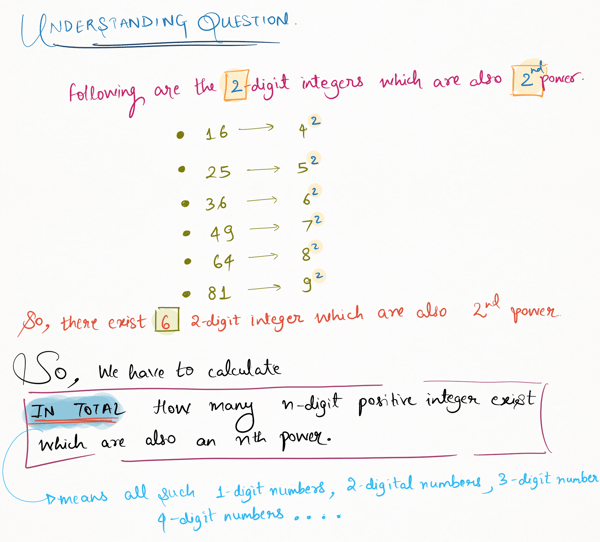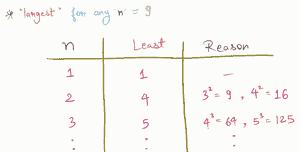Related Tags

python
project euler
communitycreator

# Project Euler 63: Powerful digit counts

Armaan Nougai

### Problem statement

How many $n$-digit positive integers exist that are also an $nth$ power?

ex => 16807 is a 5-digit number and also 7^5.### Solution approach

Any n-digit number x can be represented as follows:

10^(n-1) < x < 10^n


We will run a while loop for n from 1, and increment the result by largest-least until least > 10.

1. least is a number whose $nth$ power is the smallest n-digit $nth$ power of any number.

2. largest is a number whose $nth$ power is the largest n-digit $nth$ power of any number.

#### Example

• For n=2:
• least = 4 because $3^2$ is 9, which is 1-digit.

• largest = 9 because $10^2$ is 100, which is 3-digit.

• So, we will increment the result by largest-least+1, i.e., 9-4+1=6.from math import ceil
# ceil(x) returns least whole number greater than x

result=0
least=1
largest=9
n=1
while least<10 :
least = ceil((10**(n-1))**(1/n))

result += largest-least +1
# +1 because range of (least,largest) is inclusive
n+=1

print(result)


RELATED TAGS

python
project euler
communitycreator

CONTRIBUTOR

Armaan Nougai
RELATED COURSES

View all Courses

Keep Exploring

Learn in-demand tech skills in half the time